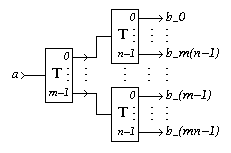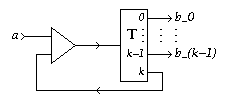EDIS: Guide | FAQ | New | Search | Bibliography | Index | Feedback

# k-Toggle

## Specifications

### Informal

A k-Toggle has one input terminal a and k output terminals b0 through bk-1. Each input signal produces one output signal. Input and output signals alternate. Signals on the output terminals are produced cyclicly, starting with b0, and then b(i+1)mod k after bi.

### XDI

Schematic diagram for a k-Toggle:[Zoom|FIG]

XDI state graph and specification for a k-Toggle with k=3:

Specification in XDI model.

. (Not available for general k)

### Verdect

Parameterized definitions are not possible in VERDECT, but this sketch gives the general idea:

Specification in Verdect:

```define TOGGLE( a?, b0!, ..,  b(k-1)! ) =
pref *[ a?; b0!; .. ; a?; b(k-1)! ]
end
```

### DI Algebra

Pure DI Algebra does not allow a generalized specification. The following specification therefore needs instantiation before interpretation:

Specification in DI Algebra:

```T(k) = T(k,0)

where

T(k,i) = a?; bi!; T(k,(i+1) mod k)
```

.
The specification of the case k=3 is as follows:

Specification in DI Algebra:

```# Generated by expexp.pl
NAME ="T"
I    = { a? }
O    = { b0!, b1!, b2! }

T_0_3 = a?; b0!; T_1_3

T_1_3 = a?; b1!; T_2_3

T_2_3 = a?; b2!; T_0_3

```

.

## Properties

XDI Report for the case k=3. (Not available for general k)

Tk(a; b0, b1..., bk-1) / a b0 = Tk(a; b1, ..., bk-1, b0)

The k-Toggle satisfies Rules Y' and Z'.

## Implementations

### DI Decompositions

1. A k-Toggle with k=1 is a Wire.
2. A k-Toggle with k=2 is a Toggle.
3. A k-Toggle can be implemented as a small state machine using a Latch by feeding output i to input (i+1) mod k (cf. Toggle Implementations).
4. For m>0 and n>0, a k-Toggle with k=mn can be implemented with one k-Toggle with k=m and m k-Toggles with k=n[Zoom|FIG]
5. For k>0, a k-Toggle with k=m can be implemented by feeding back one output, say bk, of a k-Toggle with k=m+1 with a Merge:[Zoom|FIG]
6. By suitably combining implementation schemes 2, 4 and 5 one can obtain implementations of k-Toggles for arbitrary k>0, in terms of Toggles and Merges. When k is a power of 2, only scheme 4 is needed (the result does not depend on whether k-Toggles with k=2 are introduced `on the left' or `on the right': the scheme is commutative for powers of 2 constructed in this way). The implementation of a k-Toggle with k=2^m requires 2^m-1 Toggles (no Merges).

When k is not a power of 2 (hence, k>2), also scheme 5 is needed. In this case, the resulting implementation does depend on the order. For instance, consider the implementation of a k-Toggle with k=5. One can start with a k-Toggle with k=8. to reduce it to a k-Toggle with k=5. This results in an implementation with 7 Toggles and 3 Merges.

Alternatively, we can first implement a k-Toggle with k=3 by reducing a k-Toggle with k=4 once according to scheme 5. This costs three Toggles and one Merge. Using scheme 4, the k-Toggle with k=3 can be made into a k-Toggle with k=6 by adding three Toggles `on the right'. The k-Toggle with k=6 can then be reduced to a k-Toggle with k=5 This implementation consists of 6 Toggles and 2 Merges.

Using scheme 4 to make a k-Toggle with k=6 from one Toggle (`on the left') and two k-Toggles with k=3 results in yet another implementation, costing 7 Toggles and 3 Merges.

Theorem: When constructing a k-Toggle with t Toggles and m Merges we must have k=t-m+1 and, hence, t>=k-1.

7. For m>0, n>0, and m and n relatively prime, a k-Toggle with k=mn can be implemented with one k-Toggle with k=m, one k-Toggle with k=n, and a Decision-Wait:[Zoom|FIG]

### Using Boolean Gates

No information available

### Using Transistors

No information available

## Generalizations

The k-Toggle can be further generalized to a L-Pattern Generator that cycles through an arbitrary pattern over its outputs. A k-Toggle is a (b0...bk-1)-Pattern Generator.

## Miscellaneous

In [Ebergen89, p. 140], a decomposition of a k-Toggle with k=3 is presented.

Exercise: What is an optimal implementation scheme for k-Toggles in terms of Toggles and Merges. The cost function can either be size or `average response time'.

## References

[Ebergen89, p. 140]